Explore BrainMass
Share

# Straight-Line Method of Depreciation

Straight-line depreciation is the most common method used by companies for depreciating long-term tangible assets for financial reporting. The straight-line depreciation method benefits from simplicity, since the same amount of depreciation is expenses each period. This method is also promotes matching when creeping obsolescence contributes to a constant loss of value each period from the long-term asset.

Calculating Straigh-Line Depreciation

Step 1: Calculate the depreciable amount. The depreciable amount is typically equal to the amount that the asset's book value or historical cost exceeds its estimated salvage value. An asset's book-value or historical cost is often referred to as its "cost-basis" when we are talking about depreciation.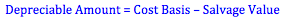Step 2: Calculate the depreciation rate. We can calculate what is know as the straight-line rate by dividing the value "1" by the number of years in the operating asset's useful life. This gives us a percentage-rate for depreciation each year. For example, imagine we purchased a van with a useful life of 4 years; under the straight-line method we would depreciate 25% of the asset's value each year. 25% is called the straight-line rate and is calculated from dividing "1" by "4", where 1/4 = 0.25.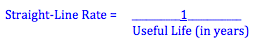Step 3: Calculate the annual depreciation expense. We can calculate the annual depreciation expense by multiplying our straight-line rate and our depreciable amount. For example, assume our van has a cost-basis of \$20,000 and a salvage value of \$4,000. We know our straight line rate is 25% from above. Our depreciable cost is \$16,000 (equal to \$20,000 - \$4,000). Our annual depreciation will therefore be \$4,000 (equal to 25% of \$4,000).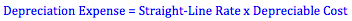Step 4: Record depreciation expense. To record depreciation, we debit an expense account for depreciation for the period and credit a contra-asset account typically called accumulated depreciation. The depreciation expense account is closed each year during the closing entries. The accumulated depreciation account is a permanent account that equals the value of all the depreciation expense recognized to date. The carrying amount of the asset is equal to the debit value of the asset in its asset account minus the credit value of accumulate depreciation in the contra asset account.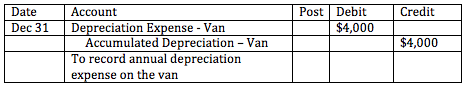When other methods of depreciation are used, the same accounts are debited and credited, but the amounts may be different.

General Formula

A general formula can be used for straight line depreciation. This formula combines our calculations in our first three steps into one formula.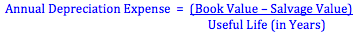### Straight-Line Method of Depreciation

#1: Using the following data and the straight-line method of depreciation, compute depreciation expense, and make the necessary journal entry to record depreciation expense for the first year. Cost of machine . . . . . . . . . . . . . . . . . . . . . . . . . . . . . . . . . . . . . . . . . . \$1,000,000 Estimated useful life

### Depreciation, straight-line method (Felix Industries)

Felix Industries purchased a grinder 5 years ago for \$15,000. It is being depreciated on a straight-line basis over 15 years to an estimated salvage value of zero. It could be sold now for \$6,000. The firm is considering selling it and purchasing a new one. The new grinder would cost \$25,000 installed and would be depreciated on

### Depreciation Expense - Straight-Line Method

Ben Gordon Corporation constructed a building at a cost of \$10,000,000. Average accumulated expenditures were \$4,000,000, actual interest was \$600,000, and avoidable interest was \$300,000. What is the depreciation expense for the first full year using the straight-line method if the salvage value is \$800,000, and the useful life

### Straight Line Depreciation and Gain or Loss on Disposition

A cutter cost \$72000 January 5, 1999 with estimated life of 10 years and salvage value of \$12,000. April 3, 2004 it was exchanged for a similar cutter with market value of \$36,000. Fenner also received \$9,000 cash. The fiscal period ended Dec 31, 2003 and used straight line depreciation. Show calculation of amount of gain or

### Straight Line depreciation

Problem: Oren Company's air-conditioning system has just completed the eight year of an estimated ten-year life. The system cost \$60,000 and now has accumulated depreciation of \$48,000. At the beginning of the ninth year, the company expects to spend \$16,000 on a complete renovation of the system and expects the total life of

### Financial Statements & Depreciation

See attached file for full problem description. Swanson & Hiller purchased a new machine on September 1, 2006 at a cost of \$108,000. The machine's estimated useful life at the time of the purchase was five years, and its residual value was \$8,000. Complete schedules assume that the half-year convention is us

### v

On January 1, 2005, Solomon Company purchased the following two machines for use in its production process. Machine A: The cash price of this machine was \$38,500. Related expenditures included: sales tax \$2,200, shipping costs \$175, insurance during shipping \$75, installation and testing costs \$50, and \$90 of oil and lubrica

### example of an asset used for the straight line depr method

Give an example of an asset that would be 'logical' to use the straight line depreciation method, and why. Then provide an example of an asset where it is more appropriate to use an accelerated method and why.

### Straight line Method of depreciation

Using the straight line method of depreciation for a 10-year period on a piece of equipment costing \$15,000 with a salvage value of 1,000, for a 10-year period, the approximate annual depreciation would be?